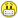+0

# How can I solve the first question ?

0
1589
19

How can I solve the first question ?and in this question I want to know if my answer is correct or no

http://i62.tinypic.com/2lkslxw.jpg

Jul 31, 2014

#14
+15

Melody is correct....no solution exists.......here's a graph of both functions....notice that there are no intersection points.....Jul 31, 2014

#1
+10

We have

1/x ≤ x         multiply both sides by x   .....note that we're assuming that x ≠ 0

1 ≤ x2          subtract x2 from both sides

1- x2 ≤ 0        I like to turn the inequaliy into an equality and factor ....this gives us some "interval" points to work with!!

(1 - x) (1 + x) = 0       Then x = ± 1

Our answer comes from two of four intervals (-∞, -1], [-1, 0), (0, 1] or [1, ∞)

If you pick a point in each interval, you'll find that [-1, 0) and [1, ∞)  are the intervals that "work' in the original inequality.......Jul 31, 2014
#2
0

why you use the zero ..؟

Jul 31, 2014
#3
+10

We aren't including 0 !!!   That's what the parentheses indicates....if we were including it, we would have had a bracket....the interval is everything from -1 up to, but not including, 0 .......Jul 31, 2014
#4
+10

Maybe I didn't look at your answer hard enough Chris but I think it has a floor in it.

If you multiply an inequality by x and x is negative then you have to turn the sign around.

Therefore you end up with 2 equations to solve.

It is better to multiply by something that you KNOW is positive.  Squared things are always positive!

Edit:    you cannot divide by 0 so    $${x\ne0}$$

$$\begin{array}{rll} \frac{1}{x}&\le&x\\\\ x^2\times\frac{1}{x}&\le&x^2 \times x\\\\ x&\le&x^3\\\\ -x^3+x&\le&0\\\\ -x(x^2-1)&\le&0\\\\ -x(x-1)(x+1)&\le&0\\\\ \end{array}$$

Now the easiest way to finish this is with a graph of

$$y=-x(x-1)(x+1)$$  and see where it intersects with $$y\le0$$

I am going to try and do a quick sketch - I know how to do a proper graph but I want to try and replicate what I would do by hand.

It is a cubic because the highest power of x is 3 and it will finish in the 2nd and 4th quads because the leading coefficient is -1I can see from the graph that the answer is   (this answer has been very slightly edited)

$$-1\lex\le x<0 \qquad or \qquad x\ge1\\ [-1,0)\;\cup \; [1,\infty)$$

I think it is right now Chris - Do we have the same answer?

Jul 31, 2014
#5
+10

Melody is correct in suggesting that I might should have reversed the signs......however, I couldn't say at that point whether x was negative or not.......all I could say originally is that x ≠ 0....it might have been that x only took on positive values...!!!! Besides, in these types of problems, I like to generally ignore the inequality signs and set up an equality that will give me some intervals to work with - which is what I did. By picking some test points in these intervals, we can determine which values make our original inequality true, and I don't have to necessarily worry about the direction of the inequality sign throughout the problem. This may not be the fastest way to solve such things, but it's the method I learned......

Here's the graph  of both functions......y = 1/x is in red     y = x is in blueNote that the intervals I have noted in my answer appear to be correct....Jul 31, 2014
#6
+5

Melody

I don't understand some points of your explain but I take your information and solve the question by this way

http://im44.gulfup.com/n3q0s0.jpg

Jul 31, 2014
#7
+5

Yes that is fine 15threefold.  It is almost the same.

I remember I did not comprehend the relevance of the graph for a long time either.

It is really good when you do get it but your method will always work well and it is almost as easy.Jul 31, 2014
#8
+5

thank you melody

what about the second question .؟

Jul 31, 2014
#9
+5

Hi 15x3

I didn't even notice that question!

$$|2x-9|&=&3-x\\\\ \begin{array}{rlllrl} 2x-9&=&3-x\qquad &or \qquad 2x-9&=&-(3-x)\\ 3x&=&12\qquad &or \qquad 2x-9&=&-3+x\\ x&=&4\qquad &or \qquad x&=&6\\ \end{array} \\BUT\\ 3-x\ge 0\\ -x\ge -3\\ x\le 3\\$$

Therefore there are no solutions.

-----------------------------------------------------------

I am just not getting the hang of this aligning properly, I wanted the x up against the equal sign, and its not there!

This is my code.

\end{array} \\

Can you try and explain please?

Jul 31, 2014
#10
+5

sory melody how do you know that  3-x bigger than 0 !

Jul 31, 2014
#11
+10

Because it is equal to an absolute value so it can't be negative!

Do you see what I mean?

Jul 31, 2014
#12
0

yes yes ... now I understand this question ..

very nice ideathank you melody

but why you call me 15×3 !

Jul 31, 2014
#13
+5

Because you are xvxvxv

xv is 15 in roman numerals and there are 3 of them so you are 15x3Jul 31, 2014
#14
+15

Melody is correct....no solution exists.......here's a graph of both functions....notice that there are no intersection points.....CPhill Jul 31, 2014
#15
+5

Hey, 15x3 if you are feeling really generous you could give CPhill and me some thumbs up.If you don't like me calling you 15x3 you'd better say so because otherwise you are stuck with it.Jul 31, 2014
#16
0

I'm sorry my friend ...

I forgot it

and i'm never get angry ... and I am grateful for you and CPhill

Ican't express my felling because English is not my mother tongueJul 31, 2014
#17
+5

No problem.....xvxvxv......English is my "mother tongue" and I'm still strugglig with it !!!!Jul 31, 2014
#18
0

HaHaHa ....

Jul 31, 2014
#19
0

Thanks 15x3.Jul 31, 2014